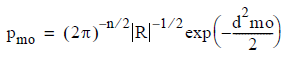# 5.4.5 - Expectation Maximization Algorithm - Teradata Warehouse Miner

## Teradata Warehouse Miner User Guide - Volume 3Analytic Functions

Product
Release Number
5.4.5
Published
February 2018
Language
English (United States)
Last Update
2018-05-04
dita:mapPath
yuy1504291362546.ditamap
dita:ditavalPath
ft:empty

The clustering algorithm requires specification of the desired number of clusters. After preprocessing, an initialization step determines seed values for the clusters, and clustering is then performed based on conditional probability and maximum likelihood principles using the EM algorithm to converge on cluster assignments that yield the maximum likelihood value.

In a Gaussian Mixture (GM) model, it is assumed that the variables being modeled are members of a normal (Gaussian) probability distribution. For each cluster, a maximum likelihood equation can be constructed indicating the probability that a randomly selected observation from that cluster would look like a particular observation. A maximum likelihood rule for classification would assign this observation to the cluster with the highest likelihood value. In the computation of these probabilities, conditional probabilities use the relative size of clusters and prior probabilities, to compute a probability of membership of each row to each cluster. Rows are reassigned to clusters with probabilistic weighting, after units of distance have been transformed to units of standard deviation of the standard normal distribution via the Gaussian distance function:where the following is true:
• p is dimensioned 1 by 1 and is the probability of membership of a point to a cluster
• d is dimensioned 1 by 1 and is the Mahalanobis Distance
• n is dimensioned 1 by 1 and is the number of variables
• R is dimensioned n by n and is the cluster variance/covariance matrix

The Gaussian Distance Function translates distance into a probability of membership under this probabilistic model. Intermediate results are saved in Teradata tables after each iteration, so the algorithm may be stopped at any point and the latest results viewed, or a new clustering process begun at this point. These results consist of cluster means, variances and prior probabilities.